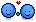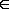# MATH TIME!

Discussion in 'User Topics' started by Mr.Lame, Jan 9, 2018.

1. ### Mr.LameVeteran

Blogs:
28
Joined:
Dec 7, 2009
Posts:
1,540
628
My brains dead i feel it, talk to you tomorrow , Thanks again Mark I super appreciate your help.

2. ### Mr.LameVeteran

Blogs:
28
Joined:
Dec 7, 2009
Posts:
1,540
628
Markkkkyyy poo @MarkFL .

x^4 − 13x^2 + 36

Last question T.T

3. I think you should start paying him

•Like x 2
4. ### Mr.LameVeteran

Blogs:
28
Joined:
Dec 7, 2009
Posts:
1,540
628
I pay in respect

•Love x 1
5. Okay, first we observe that this is a quadratic in x^2.

So, we want to look for two factors of 36 whose sum is -13, and they are -4 and -9...so we have:

x^4 - 13x^2 + 36 = (x^2 - 4)(x^2 - 9)

Now, we see both factors are differences of squares, so we have:

x^4 - 13x^2 + 36 = (x^2 - 4)(x^2 - 9) = (x^2 - 2^2)(x^2 - 3^2) = (x + 2)(x - 2)(x + 3)(x - 3)

And we're done.•Love x 2
6. ### Mr.LameVeteran

Blogs:
28
Joined:
Dec 7, 2009
Posts:
1,540
628

How the **** did you do that so fast . You rock for real though•Love x 1
7. ### Mr.LameVeteran

Blogs:
28
Joined:
Dec 7, 2009
Posts:
1,540
628
@MarkFL can you just be my tutor? Hahahaha

8. Please feel free to ask any questions here, even questions about concepts and not just actual assigned problems. I will do my best to explain.•Like x 1
9. ### Mr.LameVeteran

Blogs:
28
Joined:
Dec 7, 2009
Posts:
1,540
628
I'm going to need this more than you'd ever know!

•Like x 1
10. ### Mr.LameVeteran

Blogs:
28
Joined:
Dec 7, 2009
Posts:
1,540
628
Hey @MarkFL I do not understand how to do this question.

11. ### Mr.LameVeteran

Blogs:
28
Joined:
Dec 7, 2009
Posts:
1,540
628
List the four smallest elements of the set.
{y|y = x2 − 7, xintegers}

12. ### Mr.LameVeteran

Blogs:
28
Joined:
Dec 7, 2009
Posts:
1,540
628
x^2*

13. What's the question?

14. Nevermind...you posted it...

15. What is the smallest x^2 can be?

16. ### Mr.LameVeteran

Blogs:
28
Joined:
Dec 7, 2009
Posts:
1,540
628
Infinity? D:

17. That's the largest value it can approach as x gets farther and farther from zero. The smallest value x^2 can be is zero, and that's when x is zero. So, given the smallest value for x^2 is zero, then the smallest value for y will be -7 because y is defined to be x^2 - 7. And so given that y(x) = x^2 - 7, we have:

y(0) = 0^2 - 7 = -7

Next we observe that if we move one unit away from zero, to ±1, we find:

y(±1) = (±1)^2 - 7 = 1 - 7 = -6

Likewise:

y(±2) = (±2)^2 - 7 = 4 - 7 = -3

y(±3) = (±3)^2 - 7 = 9 - 7 = 2

And so the 4 smallest elements of the given set are {-7, -6, -3, 2}.18. ### Mr.LameVeteran

Blogs:
28
Joined:
Dec 7, 2009
Posts:
1,540
628
You lost me T.T

19. Are you familiar with parabolic curves, specifically the vertex and axis of symmetry?

•Like x 1

Blogs:
28
Joined:
Dec 7, 2009
Posts:
1,540